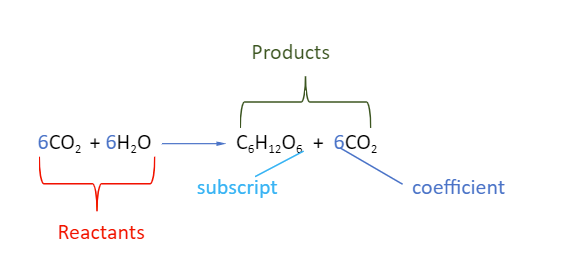• ### Browse All Lessons

##### Assign Lesson

Help Teaching subscribers can assign lessons to their students to review online!

 Tweet# Chemical Equation

This lesson aligns with Next Generation Science Standards (NGSS) PS1.B

Introduction
During a chemical reaction, the bonds of the substances that react chemically are broken, and new bonds are formed between the new substances. The substances initially involved are known as reactants, and the new substances that are formed from the reactants are known as products. For example, when a piece of wood is burned, the matter that makes up that piece of wood is converted into ash, smoke, and gases. The chemical energy that was stored in the wood is converted into thermal energy. A chemical equation shows what happens in a chemical reaction with the help of chemical formulas. In this lesson, we will discuss chemical equations and how to write a chemical equation to represent a change in a chemical reaction.

Chemical Equation
A chemical equation is a model that represents a chemical reaction. A chemical equation is a symbolic representation of a chemical change in the form of chemical formulas and other symbols. The rearrangement of atoms of the reactants in a chemical reaction cannot be directly observed, but a chemical equation helps us to understand how a chemical reaction is taking place. For example, magnesium reacts with oxygen to form magnesium oxide.

$2Mg + O_2 rarr 2MgO$

Writing a Chemical Equation
A chemical equation is a complete description of a chemical reaction. There are some rules to write a chemical equation that are listed below:

Reactants
Reactants are written on the left side of the chemical equation.

Products
Products are written on the right side of the chemical equation.

Coefficient
A coefficient is a number that is written in front of the chemical formula to show how many molecules of a compound are represented in a chemical equation. For example, in the above equation, the coefficient of MgO is 2 showing that 2 molecules of magnesium oxide are formed in the reaction.

Subscript
A subscript is written below and right to the chemical formula. It represents how many atoms of an element are taking part in a chemical reaction.

Example: Photosynthesis
During the process of photosynthesis, the sunlight is captured by plants, the carbon dioxide, and water recombine as reactants, and the stable products of this reaction are oxygen and glucose ($C_6H_12O_6$). To understand this reaction correctly, the chemical equation shows carbon dioxide and water as $CO_2$ and $H_2O$. The coefficient of carbon dioxide, water, and oxygen is 6. Only one molecule of glucose is formed; therefore, no coefficient is written in front of the chemical formula for glucose.Balancing a Chemical Equation
It is important to balance a chemical equation to fulfill the law of conservation of mass, which states that “mass is neither created nor destroyed in a chemical reaction.” Therefore, the types and number of reactants on the left side are always equal to the types and number of products on the right side in a chemical equation. For example, the following chemical equation shows the formation of water.

$2H_2 + O_2 rarr 2H_2O$

Representing the Direction in a Chemical Equation
The reactants are converted into products in a chemical reaction that can be separated by using the following symbols.
• To represent a net forward reaction, the symbol ‘→’ is used. The arrow sign shows the direction of the reaction.
• To represent a state of chemical equilibrium, the symbol ‘⇌’ is used.
• To represent a reaction that proceeds in both forward and backward directions, the symbol ‘⇄’ is used.

Representing the Physical State in a Chemical Equation
The following symbols are used to describe the physical state of the reacting entities.
• The symbol (s) shows that an entity is in a solid state
• The symbol (l) represents the liquid state
• The symbol (g) describes that atoms or molecules are in the gaseous state
• The symbol (aq) refers to an aqueous solution.

$6CO_2 (g)+ 6H_2O(l) rarr C_6H_12O_6 (aq) + 6CO_2 (g)$

Summary
• A chemical equation can describe a chemical reaction that provides complete information about how reactants are transformed into products.
• In a chemical equation, the reactants are written on the left side, whereas the products are written on the right side of an arrow.
• The number of reactants must be equal to the number of products.
• In order to represent a net forward reaction and a state of chemical equilibrium, the symbols ‘→’ and ‘⇌’ are used, respectively.
• The symbols (s), (l), and (g) are used to describe states of matter in a chemical equation.

Related Lessons: